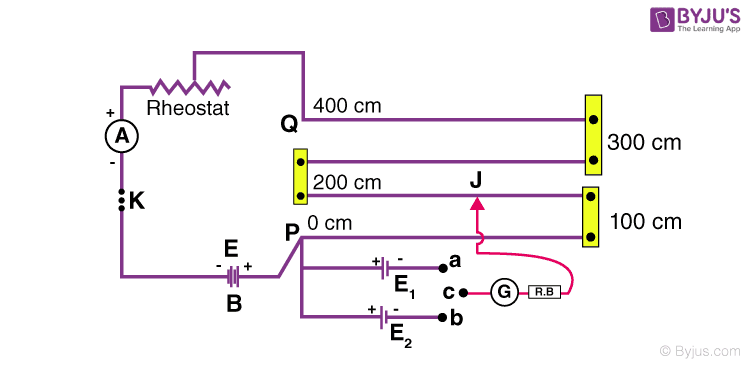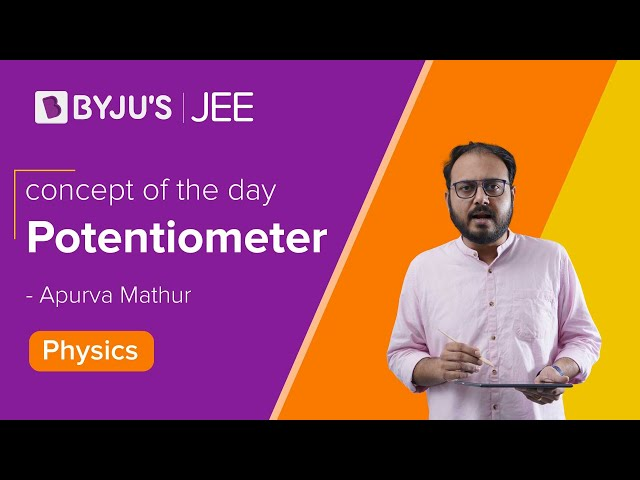# To Compare The EMF Of Two Given Primary Cells Using A Potentiometer

## Aim

To compare the EMF of two given primary cells (Daniel and Leclanche cells) with the help of a potentiometer.

## Apparatus/Material Required

• Potentiometer
• Daniel Cell
• Leclanche Cell
• low resistance Rheostat
• Ammeter
• Voltmeter
• Galvanometer
• A one-way key
• A two-way key
• Set Square
• Jockey
• Resistance Box
• Connecting wires
• Piece of sandpaper

## Theory:

Using a voltmeter, it is possible to measure only the potential difference between the two terminals of a cell, but using a potentiometer, we can determine the value of emf of a given cell. Where E1 and E2 are EMFs of two cells, l1 and l2 are the balancing lengths when E1 and E2 are connected to the circuit respectively, and φ is the potential gradient along the potentiometer wire.

E1 /E2 = φ l1 /φ l2 = l1 /l2

## Circuit Diagram## Procedure

1. Connect the circuit as shown in the figure.
2. With the help of sandpaper, remove the insulation from the ends of connecting copper wire.
3. Measure the EMF (E) of the battery and the EMFs (E1 and E2) of the cell and see if E1 > E and E2 > E.
4. Connect the positive pole of the battery to the zero ends (P) of the potentiometer and the negative pole through the one-way key, low resistance rheostat, and the ammeter to the other end of the potentiometer (Q).
5. Connect the positive poles of the cells to the terminal at the zero ends (P) and the negative poles to the terminals a and b of the two-way key.
6. Connect the common terminal c of the two-way key through a galvanometer (G) and a resistance box to the jockey J.
7. Take maximum current from the battery by making the rheostat resistance zero.
8. Insert the plugin, the one-way key through the resistance box and the galvanometer to the jockey J.
9. Take out the 2000 Ω plug from the resistance box.
10. Note down the direction of the deflection in the galvanometer by pressing the jockey at zero ends.
11. Now, press the jockey at the other end of the potentiometer wire. If the deflection is in the opposite direction to that in the first case, the connections are correct.
12. Push the jockey smoothly over the potentiometer up to a point where the galvanometer shows no deflection.
13. Put the 2000 Ω plug back into the resistance box and obtain the null point position accurately with the help of the set square.
14. Note the length l1 of the wire for the cell E1.
15. Note the current as indicated by the ammeter.
16. Disconnect the cell E1 from the plug
17. Connect E2 by inserting the plug into the gap of the two-way key.
18. Take out a 2000 ohms plug from the resistance box and slide the jockey along the potentiometer wire and obtain no deflection position.
19. Put 2000 ohms plug back in the RB and obtain null for E2.
20. Note the length L2 of wire in this position for the cell E2.
21. By increasing the current and adjusting the rheostat, we get three sets of observations.

## Observation

E.M.F of battery, E =

E.M.F of Leclanche cell, E1 =

E.M.F of Daniel cell, E2 =

Range of voltmeter =

Least count of voltmeter =

Least count of ammeter =

Zero error of ammeter =

 S. No Corrected Ammeter Reading Balance point when E1 (Leclanche cell) in the circuit l1 cm Balance point when E2 (Daniel cell) in the circuit l2 cm $$\begin{array}{l}\frac{E_1}{E_2}=\frac{l_1}{l_2}\end{array}$$ (1) (2) 1 (3a) 2 (3b) Mean l1 (3c) 1 (4a) 2 (4b) Mean l2 (4c) (5) 1 2 3

### Calculations

1. For each observation, find mean l1 and mean l2 and record it 3c and 4c respectively.
2. Find E1/E2, by dividing l1/l2
3. Find the mean of E1/E2

## Result

The ratio of EMFs, E1/E2 ≅ _____.

## Viva Voice

1. What is an EMF of a cell?

Ans: Electromotive force is the measurement of the energy that causes the current to flow through a circuit. It is also known as voltage and is measured in volts.

2. What is a potentiometer?

Ans: A potentiometer is a three-terminal device that is used to measure the potential difference by manually varying the resistance.

3. On what principle does the potentiometer work?

Ans: For a constant current, the fall of the potentiometer along a uniform wire is directly proportional to its length.

4. How is the emf of the cell determined for given cells?

Ans: E1/E2 = l1/l2

5. Is the cross-section of the potentiometer wire uniform?

Ans: NoStay tuned to BYJU’S and Fall in Love with Learning!

Test Your Knowledge On To Compare The Emf Of Two Given Primary Cells Using Potentiometer Experiment!